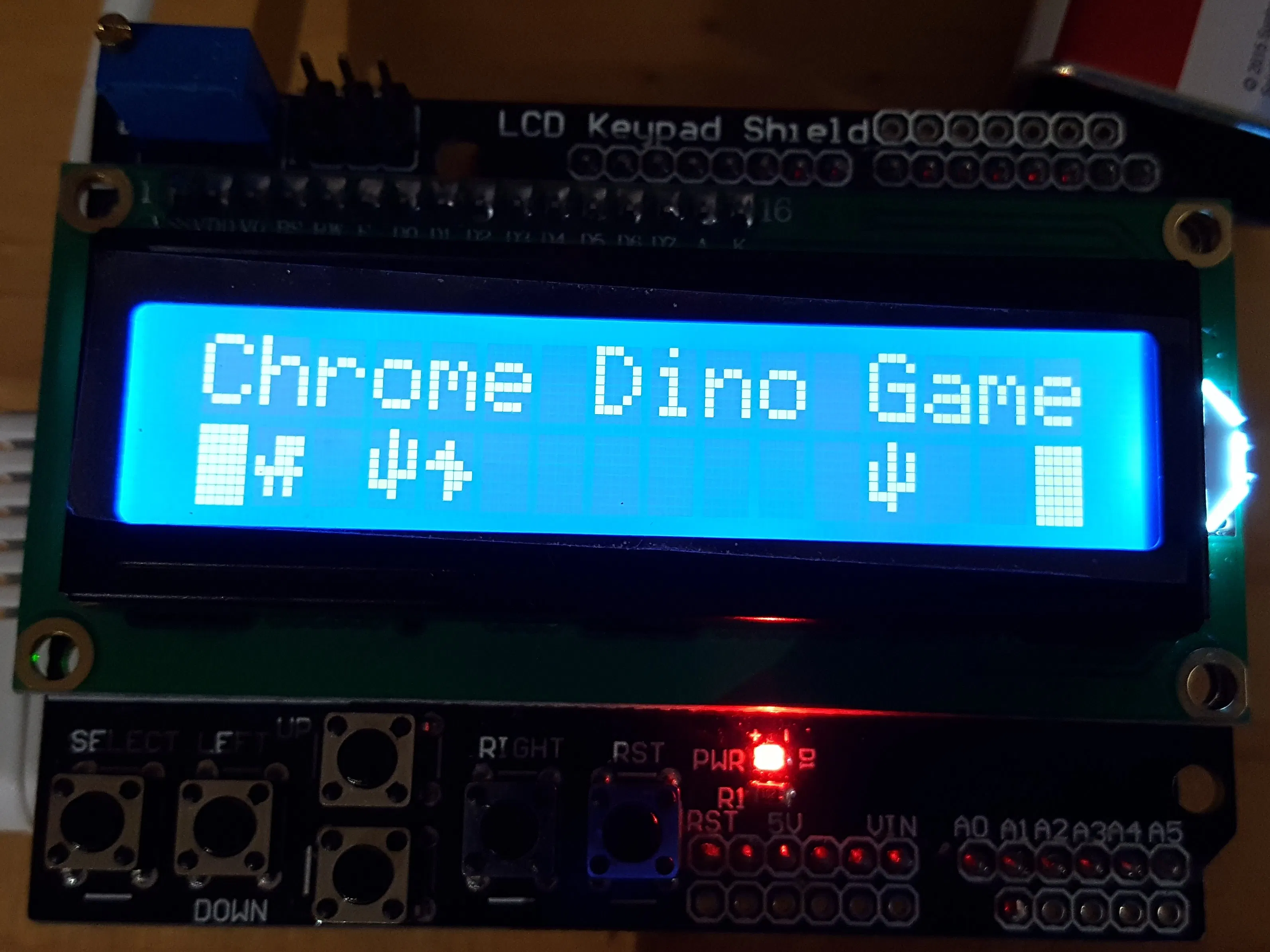# The Chrome Dino Game on an LCD Shield

The Chrome dinosaur game that is now on an Arduino LCD shield!

IntermediateFull instructions provided10,210## Things used in this project

### Hardware componentsArduino UNO & Genuino UNO Any Arduino that follows the Arduino 1.0 pinout should work
×1
 Kuman LCD Shield If you are using a different shield, do not forget to change the pins used by the lcd and the button definitions!
×1
 Knowledge on operating your computer and the Arduino IDE
×1

### Software apps and online servicesArduino IDE Do I really need to mention this though?

### Hand tools and fabrication machines

 Hands
 Computer

## Schematics

### Schematic

It is a bare Arduino. Pretend there is a LCD Shield attached to the Arduino

## Code

### Chrome_Dino_Game_on_LCD

C/C++
Paste into editor as main sketch
```#include <LiquidCrystal.h>
#include <EEPROM.h>

#include "bitmaps.h"

LiquidCrystal lcd(8, 9, 4, 5, 6, 7);

#define btnRIGHT  0
#define btnUP     1
#define btnDOWN   2
#define btnLEFT   3
#define btnSELECT 4
#define btnNONE   5

byte runnerArea {32, 32, 32, 32, 32, 32, 32, 32, 32, 32, 32, 32, 32, 32, 32, 32};
byte jump = 32;

int score = 0;
bool freeze_score = 0;

byte correct_code = 123;

unsigned long previousMillis = 0;
unsigned long previousMillisLED = 0;
unsigned long jumpTime = 0;
const int jumpLength = 500;

#define checkSafe runnerArea == 32 || runnerArea == 0

const byte chance_of_ob = 15;
int speedOfScroller = 300;

void setup() {
lcd.begin(16, 2);
Serial.begin(9600);

lcd.createChar(0, dino);
lcd.createChar(1, cacti);
lcd.createChar(2, bird);
lcd.createChar(3, block);
pinMode(A0, INPUT);
pinMode(A1, INPUT);
pinMode(13, OUTPUT);
randomSeed(A1);
lcd.clear();
showSplashScreen(1000, true);
}

void loop() {
unsigned long currentMillis = millis();
unsigned long currentMillisOb = millis();
if (currentMillisOb - previousMillis >= speedOfScroller) {
previousMillis = currentMillisOb;
if (random(chance_of_ob) == 0) {
runnerArea = 1;
} else if (random(chance_of_ob) == 1) {
runnerArea = 2;
} else {
runnerArea = 32;
}
for (int i = 0; i <= 15; i++) {
runnerArea[i] = runnerArea[i + 1];
}
if (freeze_score == 0) {
score++;
}
}
drawBarrier();

// runnerArea = 32;
if (runnerArea != 32 && (runnerArea != 1 || runnerArea != 2)) {
runnerArea = 32;
}
jump = 0;
freeze_score = 1;
jumpTime = millis();
}
if (millis() - jumpTime >= jumpLength) {
if (checkSafe) {
runnerArea = 0;
jump = 32;
freeze_score = 0;
} else {
showCrashScreen();
}
}
updateLcd();
printScore();

if (millis() - previousMillisLED >= 500) {
previousMillisLED = currentMillis;
}
}
```

### Functions

C/C++
Upload as a tab named Functions
```// Functions for main.cpp

if (adc_key_in > 1000) return btnNONE;

if (adc_key_in < 10)  return btnRIGHT;
if (adc_key_in < 110)  return btnUP;
if (adc_key_in < 300)  return btnDOWN;
if (adc_key_in < 450)  return btnLEFT;
if (adc_key_in < 700)  return btnSELECT;

return btnNONE;
}

void updateLcd() {
for (int i = 0; i <= 15; i++) {
lcd.setCursor(i, 1);
lcd.write(runnerArea[i]);
}
lcd.setCursor(1, 0);
lcd.write(jump);
}

void drawBarrier() {
runnerArea = 3;
runnerArea = 3;
}

void printScore() {
lcd.setCursor(4, 0);
lcd.print("Score: ");
lcd.setCursor(11, 0);
lcd.print(score);
}

void showSplashScreen(int delayTime, boolean wait_for_start) {
lcd.home();
lcd.print("Chrome Dino Game");
drawSplashGraphics();
delay(delayTime);
if (wait_for_start) {
checkForEEPROMUserInitErase();
}
}
lcd.clear();
}

boolean checkForEEPROMUserInitErase() {
lcd.clear();
lcd.home();
lcd.print("Enter Executive");
lcd.setCursor(0, 1);
lcd.print("Code: ");
lcd.clear();
lcd.home();
lcd.print("Chrome Dino Game");
drawSplashGraphics();
return 0;
}
lcd.home();
lcd.print("Clearing EEPROM");
EEPROMWriteInt(0, 0);
delay(250);
lcd.home();
lcd.print("               ");
lcd.home();
lcd.print("Done!");
delay(100);
delay(400);
lcd.setCursor(0, 1);
lcd.print("                ");
lcd.home();
lcd.print("Chrome Dino Game");
drawSplashGraphics();
}
return 1;
}

bool leave = 0;
byte code = 0;

lcd.setCursor(0, 1);
lcd.print("Code: ");
while (leave == 0) {
lcd.setCursor(6, 1);
lcd.print("    ");
lcd.setCursor(6, 1);
lcd.print(code);
code++;
delay(50);
} else if (read_LCD_buttons() == btnDOWN) {
code--;
delay(50);
}
if (code == correct_code) {
leave = 1;
} else {
wrong();
}
}
delay(50);
}
delay(250);
lcd.home();
lcd.clear();
lcd.print("Access granted!");
delay(500);
lcd.clear();
return 1;
}

void wrong() {
lcd.setCursor(0, 1);
lcd.print("Incorrect!");
code = 0;
delay(250);
lcd.setCursor(0, 1);
lcd.print("Code: ");
}

void drawRandChar() {
lcd.setCursor(random(3, 15), 1);
lcd.write(byte(random(1, 3)));
}

void drawSplashGraphics() {
lcd.setCursor(0, 1);
lcd.write(byte(3));
lcd.setCursor(15, 1);
lcd.write(byte(3));
lcd.setCursor(1, 1);
lcd.write(byte(0));
drawRandChar();
drawRandChar();
drawRandChar();
}

void showCrashScreen() {
lcd.setCursor(4, 1);
lcd.print("Game Over!");
delay(2500);
lcd.setCursor(4, 1);
lcd.print("Best: ");
lcd.setCursor(10, 1);
lcd.print("      ");
lcd.setCursor(10, 1);
EEPROMWriteInt(0, score);
}
while (true) {
delay(500);
}
}

{
byte two = (value & 0xFF);
byte one = ((value >> 8) & 0xFF);

}

{

return ((two << 0) & 0xFFFFFF) + ((one << 8) & 0xFFFFFFFF);
}
```

### bitmaps.h

Upload as a seperate tab named bitmaps.h
```// Bitmaps-Do not modify

byte dino = {
0b00000,
0b00111,
0b00111,
0b10110,
0b11111,
0b01010,
0b01010,
0b00000
};

byte cacti = {
0b00100,
0b00101,
0b10101,
0b10101,
0b10111,
0b11100,
0b00100,
0b00000
};

byte bird = {
0b00000,
0b00100,
0b01100,
0b11110,
0b00111,
0b00110,
0b00100,
0b00000
};

byte block = {
0b11111,
0b11111,
0b11111,
0b11111,
0b11111,
0b11111,
0b11111,
0b11111
};
```

## Credits

### Unsigned_Arduino

0 projects • 1 follower